# EXERCISE  7.2

QUESTION 1

Which congruence criterion do you use in the following ?

(a) Given: AC = DF, AB = DE, BC = EF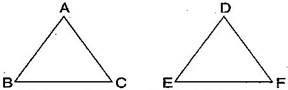Sol :

By SSS congruence criterion, since it is given that AC = DF, AB = DE, BC = EF

The three sides of one triangle are equal to the three corresponding sides of another triangle.

So , ΔABC≅ΔDEF

(b) Given: RP = ZX , RQ = ZY , ∠PRQ = ∠XZY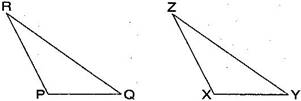Sol :

By SAS congruence criterion, since it is given that RP = ZX, RQ = ZY and PRQ = XZY

The two sides and one angle in one of the triangle are equal to the corresponding sides and the angle of other triangle.

So, ΔPQR≅ΔXYZ

(c) Given: MLN = FGH, NML = HFG, ML = FG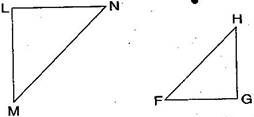Sol :

By ASA congruence criterion, since it is given that MLN = FGH, NML = HFG, ML = FG.

The two angles and one side in one of the triangle are equal to the corresponding angles and side of other triangle.

So, ΔLMN ΔGFH

(d) Given: EB = BD, AE = CB, A = C = 90°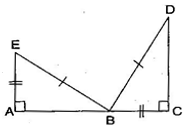Sol :

By RHS congruence criterion, since it is given that EB = BD, AE = CB, A = C = 90∘

Hypotenuse and one side of a right angled triangle are respectively equal to the hypotenuse and one side of another right angled triangle.

So, ΔABEΔCDB

QUESTION 2

You want to show that ΔART ≅ ΔPEN:

(a) If you have to use SSS criterion, then you need to show:

(i) AR = (ii) RT = (iii) AT =

Sol : (a) Using SSS criterion, ΔART ΔPEN

(i) AR = PE  (ii) RT = EN  (iii) AT = PN

(b) If it is given that ∠T = ∠N and you are to use SAS criterion, you need to have:

(i) RT =  (ii) PN =

Sol : (b) Given: T = N

Using SAS criterion, ΔART ΔPEN

(i) RT = EN (ii) PN = AT

(c) If it is given that AT = PN and you are to use ASA criterion, you need to have:

(i) ? (ii) ?

Sol : (c) Given: AT = PN

Using ASA criterion, ΔART  ≅ ΔPEN

(i) RAT = EPN   (ii) RTA = ENP

QUESTION 3

You have to show that ΔAMP ≅ ΔAMQ. In the following proof, supply the missing reasons: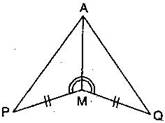Sol :

Steps Reasons
1. PM = QM 1.  Given
2.∠PMA = QMA 2. Given
3. AM = AM 3. Common
4. ΔAMP ≅ ΔAMQ 4. SAS congruence rule

QUESTION 4

In ΔABC, ∠A = 30º, ∠B = 40º and ∠C = 110º

In ΔPQR, ∠P = 30º, ∠Q = 40º and ∠R = 110º

A student says that ΔABC ΔPQR by AAA congruence criterion. Is he justified? Why or why not?

Sol :

No, because the two triangles with equal corresponding angles need not be congruent. In such a correspondence, one of them can be an enlarged copy of the other.

QUESTION 5

In the figure, the two triangles are congruent. The corresponding parts are marked. We can write ΔRAT  ?Sol :

It can be observed that ,

∠RAT = ∠WON (Given)

AR = OW (Given)

∠ART = ∠OWN (Given)

By using ASA congruence criteria ,  ΔRAT = ΔWON

QUESTION 6

Complete the congruence statement:ΔBCA  ? and  ΔQRS  ?

Sol :

Given that BC = BT

TA = CA

BA is common

∴ ΔBCA ≅ ΔBTA

Similarly PQ = RS

TQ = QS

PT = QR

∴ ΔQRS ≅ ΔTPQ

ALTERNATE METHOD

In ΔBAT and ΔBAC, given triangles are congruent so the corresponding parts are:

B, A  A, T  C

Thus, ΔBCA ΔBTA [By SSS congruence rule]

In ΔQRS and ΔTPQ, given triangles are congruent so the corresponding parts are:

R, T  Q, Q  S

Thus, ΔQRS ΔTPQ [By SSS congruence rule]

QUESTION 7

In a squared sheet, draw two triangles of equal area such that:

(i) the triangles are congruent.

(ii) the triangles are not congruent.

What can you say about their perimeters?

Sol :

(i)These triangles are congruent, i.e., ΔABC ΔPQR  [By SSS congruence rule]

Here, ΔABC and ΔPQR have the same area and the perimeter of both the triangles will be the same because they are congruent .

(ii)Here, the two triangles have the same height and base. Thus, their areas are equal. However, these triangles are not congruent to each other. Also, the perimeter of both the triangles will not be the same.

QUESTION 8

Draw a rough sketch of two triangles such that they have five pairs of congruent parts but still the triangles are not congruent.

Sol :

Let us draw two triangles ΔPQR and ΔABC.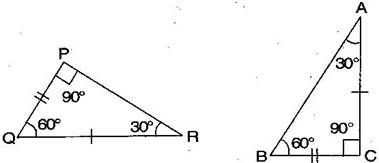All angles are equal, two sides are equal except one side. Hence, ΔPQR are not congruent to ΔABC.

QUESTION 9

If ΔABC and ΔPQR are to be congruent, name one additional pair of corresponding parts. What criterion did you use?

Sol :ΔABC and ΔPQR are congruent. Then one additional pair isGiven: B = Q = 90º

C = RTherefore, ΔABC ΔPQR [By ASA congruence rule]

QUESTION 10

Explain, why ΔABC ΔFEDSol :

Given that , ∠ABC = ∠FED …(i)

∠BAC = ∠EFD  …(ii)

The two angles of ΔABC are equal to the two respective angles of ΔFED . Also , the sum of all interior angles of a triangle is 180º . Therefore , third angle of both triangles will also be equal in measure .

∠BCA = EDF …(iii)

Also , given that BC = ED …(iv)

by using equation (i) , (ii) , (iii) and (iv) , we obtain

ΔABC≅ΔFED  (ASA criteria)

Insert math as
$${}$$